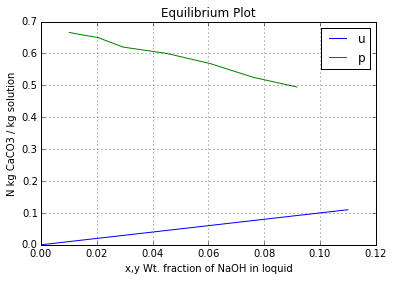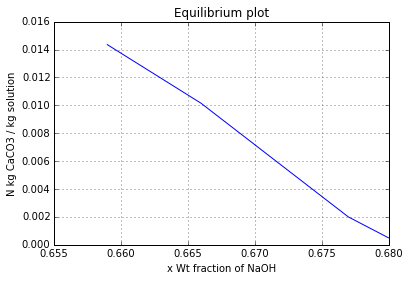# Chapter 13: Leaching¶

## Ex13.1: Page 722¶

In :
# Illustration 13.1
# Page: 722

print'Illustration 13.1 - Page: 722\n\n'

# Solution
import numpy as np
import math
#***Data***#
Density_L = 1137.0;# [kg/cubic m]
Density_S = 960.0;# [kg/cubic m]
Density_p = 1762.0;# [kg/cubic m]
A_prime = 16.4;# [square m/kg]
g = 9.81;# [square m/s]
sigma = 0.066;# [N/m]
Z = 3.0;# [m]
dia = 1.0;# [m]
#**********#

e = 1-(Density_S/Density_p);# [fraction void]
ap = A_prime*Density_S;# [square m/cubic m]
# By Eqn. 6.67:
dp = 6*(1-e)/ap;# [m]
# By Eqn. 13.6:
K = dp**2*e**3.0*g/(150.0*(1-e)**2);# [cubic m/s]
check = K*Density_L*g/(g*sigma);
if (check<0.02):
# By Eqn. 13.3:
So = 0.075;
else:
# By Eqn. 13.4:
So = 0.0018/(check)

# By Eqn. 13.2:
ZD = (0.275/g)/((K/g)**0.5*(Density_L/sigma));# [m]
# By Eqn. 13.1:
Sav = ((Z-ZD)*So/Z)+(ZD/Z);
# VolRatio=Vol liquid retained/Vol bed.
VolRatio = Sav*e;
print"Vol liquid retained/Vol bed : ",round(VolRatio,4)," cubic m/cubic m\n"
Mass = VolRatio*math.pi*dia**2*Z*Density_L/4;# [kg]
# Mass ratio=Mass Liquid/Mass dry solid
MassRatio = VolRatio*Density_L/(Density_S);
print"Mass liquid/Mass dry solid: ",round(MassRatio,4)," kg/kg\n"

Illustration 13.1 - Page: 722

Vol liquid retained/Vol bed :  0.058  cubic m/cubic m

Mass liquid/Mass dry solid:  0.0687  kg/kg



## Ex13.2: Page 749¶

In :
# Illustration 13.2
# Page: 749

print'Illustration 13.2 - Page: 749\n\n'

# Solution
import matplotlib.pyplot as plt
%matplotlib inline

#***Data***#
# Eqb=[x(Wt fraction NaOH in clear solution) N(kg CaCO3/kg soln in settled sludge) y*(wt fraction NaOH in soln of settled sludge)]
# a=H2O b=CaCO3 c=NaOH
Eqb = np.array([[0.090 ,0.495, 0.0917],[0.0700, 0.525, 0.0762],[0.0473, 0.568, 0.0608],[0.0330, 0.600, 0.0452],[0.0208, 0.620, 0.0295],[0.01187 ,0.650, 0.0204],[0.00710, 0.659, 0.01435],[0.00450, 0.666, 0.01015]]);
def f80(x):
return x
x = np.arange(0,0.12,0.01);
Mass_c = 0.1;# [kg]
Mass_b = 0.125;# [kg]
Mass_a = 0.9;# [kg]
#**************#

plt.plot(x,f80(x),label="N Vs x")
plt.plot(Eqb[:,2],Eqb[:,1],label="N Vs Y");
plt.grid('on');
plt.xlabel("x,y Wt. fraction of NaOH in loquid");
plt.ylabel("N kg CaCO3 / kg solution");
plt.legend('upper left');
plt.title("Equilibrium Plot")
plt.show()
# Basis: 1 kg soln in original mixture.
# As in Fig. 13.27 (Pg 750)
# The original mixture corresponds to M1:
NM1 = 0.125;# [kg CaCO3/kg soln]
yM1 = 0.1;# [kg NaOH/kg solution]
# The tie line through M1 is drawn. At point E1 representing the settled sludge:
N1 = 0.47;# [kg CaCO3/kg soln]
y1 = 0.100;# [kg NaOH/kg solution]
E1 = Mass_b/N1;# [kg soln. in sludge]
Ro = 1-E1;# [kg clear soln drawn]

# Stage 2:
xo = 0;# [kg NaOH/kg soln]
# By Eqn. 13.11:
M2 = E1+Ro;# [kg liquid]
# By Eqn. 13.12:
NM2 = Mass_b/(E1+Ro);# [kg CaCO3/kg soln]
# M2 is located on line RoE1. At this value of N, and the tie line through M2 is drawn. At E2:
N2 = 0.62;# [kg CaCO3/kg soln]
y2 = 0.035;# [kg NaOH/kg solution]
E2 = Mass_b/N2;# [kg soln. in sludge]
Ro = 1-E2;# [kg clear soln drawn]

# Stage 3:
xo = 0;# [kg NaOH/kg soln]
# By Eqn. 13.11:
M3 = E2+Ro;# [kg liquid]
# By Eqn. 13.12:
NM3 = Mass_b/M3;# [kg CaCO3/kg soln]
# Tie line E3R3 is located through M3.At E3:
N3 = 0.662;# [kg CaCO3/kg soln]
y3 = 0.012;# [kg NaOH/kg solution]
# By Eqn. 13.8:
E3 = Mass_b/N3;# [kg soln. in sludge]
print"The fraction of original NaOH in the slurry: ",round(E3*y3/Mass_c,4)," \n"

Illustration 13.2 - Page: 749The fraction of original NaOH in the slurry:  0.0227



## Ex13.3: Page 754¶

In :
# Illustration 13.3
# Page: 754

print'Illustration 13.3 - Page: 754\n\n'

# Solution (a)
import numpy as np
from scipy import interp
import matplotlib.pyplot as plt
%matplotlib inline
#***Data***#
# a=H2O b=CaCO3 c=NaOH
mass_c = 400;# [kg/h]
x1 = 0.1;# [wt fraction NaOH in overflow]
#**********#

Mb = 100.0;# [kg/kmol]
Mc = 40.0;# [kg/kmol]
rate_c = mass_c/Mc;# [kmol/h]
rate_b = rate_c/2;# [kmol/h]
mass_b = rate_b*Mb;# [kg/h]
# After trial calculations:
y3 = 0.01;# [kg NaOH/kg solution]
N3 = 0.666;# [kg CaCO3/kg solution]
E3 = mass_b/N3;# [kg/h]
lost_c = E3*y3;# [kg/h]
sludge_a = E3-lost_c;# [kg/h]
overflow_c = mass_c-lost_c;# [kg NaOH/kg solution]
R1 = overflow_c/x1;# [kg overflow/h]
R1_a = R1-overflow_c;# [kg/h]
RNpPlus1 = R1_a+sludge_a;# [kg/h]
# For purpose of calculation, it may be imagined that agitators are not present in the flowsheet and the first thickner is fed with the dry mixture of the reaction products, CaCO3 and NaOH, together with overflow from the second thickner.
F = 400.0;# [kg NaOH/h]
NF = mass_b/F;# [kg CaCO3/kg NaOH]
yF = 1.0;# [wt fraction NaOH in dry solid, CaCO3 free basis]
# Points R1, E3, RNpPlus1 and F are plotted as in Fig 13.30 (Pg 755) and locate the point deltaR at the intersection of lines FR1 and E3RNpPlus1 extended. The coordinates of point deltaR are NdeltaR=-0.1419, ydeltaR=-0.00213. Further computation must be done on enlarged section of the equilibrium diagram (Fig 13.31 (Pg 755)). Point deltaR is plotted and the stages stepped off in a usual manner. The construction are projected on the xy diagram. Three stages produce a value:     y3=0.001
print"The NaOH lost in sludge: ",round((lost_c/mass_c)*100,2),"%\n"
print"\n"

# Solution (b)
#*** Data***#
lost_c = 0.001*mass_c;# [kg/h]
#***********#

NNp_by_yNp = mass_b/lost_c;# [kg CaCO3/kg NaOH in final sludge]
# In order to determine the liquid content of the final sludge:
# Eqb=[N y_star]
Eqb = np.array([[0.659 , 0.01435],[0.666, 0.01015],[0.677, 0.002],[0.679, 0.001],[0.680 ,0.0005]]);
N_by_ystar = zeros(5);
for i in range(0,5):
N_by_ystar[i] = Eqb[i,0]/(Eqb[i,1]);

plt.plot(Eqb[:,0],Eqb[:,1]);
plt.grid('on');
plt.xlabel("x Wt fraction of NaOH");
plt.ylabel("N kg CaCO3 / kg solution");
plt.title("Equilibrium plot")
# By Interpolation, for N_by_ystar=NNp_by_yNp:
NNp = interp(NNp_by_yNp,N_by_ystar,Eqb[:,0]);# [kg CaCO3/kg soln]
yNp = NNp/NNp_by_yNp;# [wt fraction NaOH in the liquid of the final sludge]
ENp = mass_b/NNp;# [kg/h]
ENp_a = ENp-lost_c;# [kg/h]
overflow_c = mass_c-lost_c;# [kg/h]
R1 = overflow_c/0.1;# [kg/h]
R1_a = R1-overflow_c;# [kg/h]
RNpPlus1 = R1_a+sludge_a;# [kg/h]
# On the operating diagram (Fig 13.32 (Pg 757)) point deltaR is located and stages were constructed.
# Beyond the fourth stage, the ratio of the overflow to the liquid in the sludge become substantially constant.
R_by_E = RNpPlus1/ENp;
# This is the initial slope of the operating line on the lower part of the figure.
# From Illustration 13.2:
m = 0.01015/0.00450;
Value1 = R_by_E/m;
xNpPlus1 = 0;# [kg NaOH/kg solution]
y4 = 0.007;# [wt fraction NaOH in the liquid]
Value2 = (yNp-(m*xNpPlus1))/(y4-(m*xNpPlus1));
# From Fig 5.16: (Pg 129):
# An Additional 2.3 stages beyond 4 are computed graphically are required.
# An additional two stage will make yNp/y4=0.099:
yNp = 0.099*y4;# [wt fraction NaOH in the liquid]
print round(yNp*ENp,2),"kg NaOH was lost if 6 thickners were used\n"
# An additional three stage will make yNp/y4=0.0365:
yNp = 0.0365*y4;# [wt fraction NaOH in the liquid]
print round(yNp*ENp,3),"kg NaOH was lost if 7 thickners were used\n"

Illustration 13.3 - Page: 754

The NaOH lost in sludge:  1.88 %

0.51 kg NaOH was lost if 6 thickners were used

0.188 kg NaOH was lost if 7 thickners were used## Ex13.4: Page 758¶

In :
# Illustration 13.4
# Page: 758

print'Illustration 13.4 - Page: 758\n\n'

# Solution
import numpy
from scipy import interp
#***Data***#
# a:oil b:soyabean c:hexane
# Data=[100y*(Wt % oil in soln) 1/N(kg soln retained/kg insoluble solid)]
Data = numpy.array([[0 ,0.58],[20 ,0.66],[30 ,0.70]]);
# Soyabean feed:
percent_b = 20.0;# [soluble]
yF = 1.0;# [mass fraction oil,solid free basis]
# Solvent:
RNpPlus1 = 1.0;# [hexane,kg]
xNpPlus1 = 0;# [mass fraction oil]
# Leached Solids:
leached = 0.005;# [fraction of oil to be leached]
# Miscella:
percent_miscella = 10.0;# [percent of insoluble solid]
#**********#

N = zeros(3);
ystar_By_N = zeros(3);
for i in range(0,3):
N[i] = 1/Data[i,1];# [kg insoluble solid/kg soln retained]
ystar_By_N[i] = Data[i,0]/(100*N[i]);# [kg oil/kg insoluble solid]

# Basis: 1 kg flakes introduced
# Soyabean feed:
mass_b = 1-(percent_b/100.0);# [insoluble,kg]
F = 1.0-mass_b;# [kg]
NF = mass_b/F;# [kg insoluble solid/kg oil]

# Leached Solids:
Ratio = leached/(1-leached);# [kg oil/kg insoluble solid]
# By interpolation:
Np = interp(Ratio,ystar_By_N,N);
miscella_b = (percent_miscella/100.0)*mass_b;# [Insoluble solid lost to miscella,kg]
leached_b = (1-(percent_miscella/100.0))*mass_b;# [Insoluble solid in miscella,kg]
ENp = leached_b/Np;# [kg soln retained]
retained_a = Ratio*leached_b;# [oil retained,kg]
retained_c = ENp-retained_a;# [Hexane retained,kg]
yNp = retained_a/ENp;# [mass fraction of oil in retained liquid]

# Miscella:
mass_c = 1.0-retained_c;# [kg]
mass_a = F-retained_a;# [kg]
R1 = mass_c+mass_a;# [clear miscella,kg]
x1 = mass_a/R1;# [mass fraction of oil in the liquid]
NR1 = miscella_b/R1;# [kg insoluble solid/kg soln]

# The operating diagram is shown in Fig 13.33 (Pg 759).
# Point R1 represents the cloudy miscella and is therefore is displaced from the axis of he graph at NR1. Point deltaR is located as usual and the stages determined with the N=0 axis for all the stages but the first.
print"Between 4 and 5 stages are required\n"

Illustration 13.4 - Page: 758

Between 4 and 5 stages are required